Demo problem: Flow in a 2D channel with an oscillating leaflet

In this example we consider the flow in a 2D channel which is partially obstructed by an oscillating leaflet. We consider the case where the motion of the leaflet is prescribed – this is a "warm-up exercise" for the corresponding FSI problem in which the leaflet is an elastic structure.

# The Problem

The figure below shows a sketch of the problem: A 2D channel of height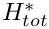and length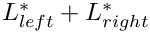is partially occluded by a (zero-thickness) leaflet of height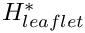. The leaflet is parametrised by a Lagrangian coordinate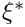so that the position vector to a material point on the leaflet is given by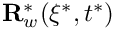, and we assume that the leaflet performs time-periodic oscillations with period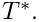Steady Poiseuille flow with average velocity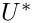is imposed at the left end of the channel while we assume that the outflow is parallel and axially traction-free.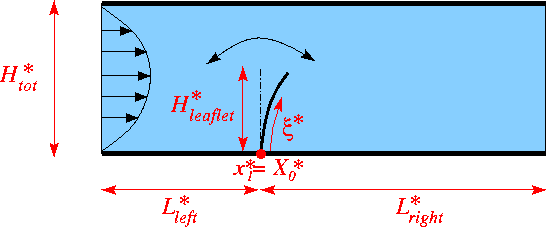Sketch of the problem in dimensional

We non-dimensionalise all length and coordinates on the channel width,, time on the natural timescale of the flow,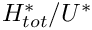, the velocities on the mean velocity,, and the pressure on the viscous scale. The problem is then governed by the non-dimensional Navier-Stokes equations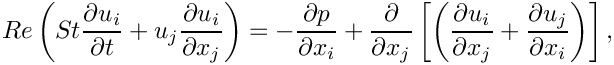where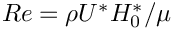and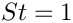, and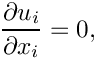subject to parabolic inflow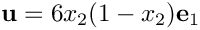at the inflow cross-section; parallel, axially-traction-free outflow at the outlet; and no-slip on the stationary channel walls,. The no-slip condition on the leaflet is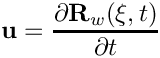and the leaflet performs oscillations with non-dimensional period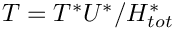. Here is a sketch of the non-dimensional version of the problem: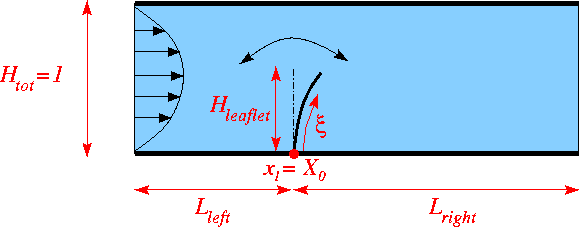Sketch of the problem in dimensionless variables.

An interesting feature of this problem is that even though the leaflet is assumed to have negligible thickness its presence will generate a pressure jump between its two faces. (The velocities are continuous because the no-slip condition imposes the same velocity on both faces.) When discretising the problem with QTaylorHood elements (for which the pressure varies continuously across the element boundaries), the mesh must therefore be "opened up" with a cut along the position of the leaflet. This is done in the constructor of the ChannelWithLeafletMesh which forms the basis of RefineableAlgebraicChannelWithLeafletMesh used to discretise this problem. (See Further comments and exercises for a more detailed discussion of the mesh.)

# Results

The figure below shows a snapshot of the flow field
(pressure contours and instantaneous streamlines) for a Reynolds number of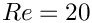and an oscillation period of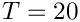, as well as the corresponding fluid mesh. Note how oomph-lib's automatic mesh adaptation has refined the mesh near the tip of the leaflet where the pressure has a singularity.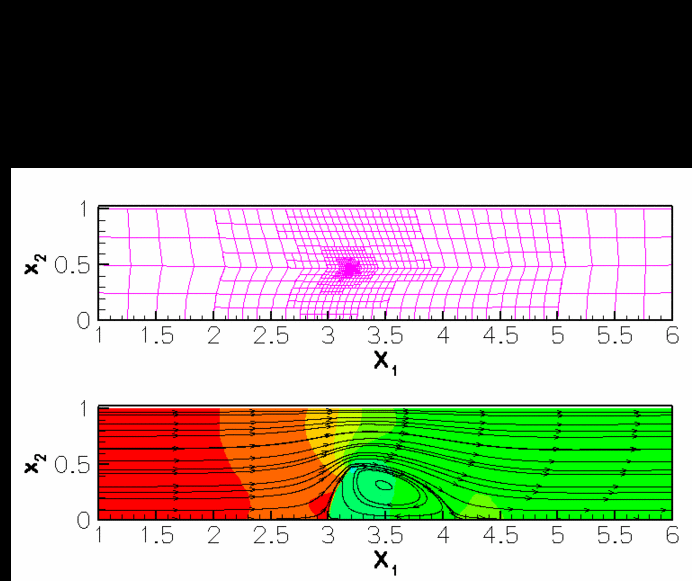Mesh (top) and flow field (bottom).

The corresponding animation illustrates the algebraic node update strategy (implemented with an AlgebraicMesh, discussed in more detail in another tutorial) and the evolution of the flow field. Note that the instantaneous streamlines intersect the (impermeable) leaflet because the leaflet is not stationary.

# The global parameters

As usual we use a namespace to define the (single) global parameter, the Reynolds number.

//==start_of_global_parameters=======================================
/// Global parameters
//===================================================================
{
/// Reynolds number
double Re=20.0;
} // end_of_namespace
//////////////////////////////////////////////////////////////////// ////////////////////////////////...

# Specification of the leaflet geometry

We specify the leaflet geometry and its time-dependent motion by representing it as a GeomObject. The GeomObject has one Lagrangian and two Eulerian coordinates, and its geometry is characterised by its length, the x-coordinate of its origin,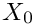, and the period and amplitude of the horizontal and vertical tip deflection.

//===start_of_leaflet_class===========================================
/// GeomObject representing a vertical leaflet that performs
/// bending and stretching oscillations.
//====================================================================
class Leaflet : public GeomObject
{
public:
/// Constructor: Pass length (in Lagrangian coordinates),
/// the amplitude of the horizontal and vertical deflection of the tip,
/// the x-coordinate of the origin and the period of the oscillation.
/// Passes the number of Lagrangian and Eulerian coordinates to the
/// constructor of the GeomObject base class.
Leaflet(const double& length, const double& d_x,const double& d_y,
const double& x_0, const double& period, Time* time_pt)
: GeomObject(1,2), Length(length), D_x(d_x), D_y(d_y), X_0(x_0),
T(period),Time_pt(time_pt) {}
/// Destructor -- emtpy
virtual ~Leaflet(){}
/// Position vector, r, to the point identified by
/// its 1D Lagrangian coordinate, xi (passed as a 1D Vector) at discrete time
/// level t (t=0: present; t>0: previous).
void position(const unsigned& t, const Vector<double>& xi,
Vector<double>& r) const
{
using namespace MathematicalConstants;
//Position
r = X_0 + D_x*xi*xi/Length/Length*sin(2.0*Pi*Time_pt->time(t)/T);
r = xi*(1.0+D_y/Length*0.5*(1.0-cos(4.0*Pi*Time_pt->time(t)/T)));
}
/// Steady version: Get current shape
void position(const Vector<double>& xi, Vector<double>& r) const
{
position(0,xi,r);
}
/// Number of geometric Data in GeomObject: None.
unsigned ngeom_data() const {return 0;}
/// Length of the leaflet
double length() { return Length; }
/// Amplitude of horizontal tip displacement
double& d_x() {return D_x;}
/// Amplitude of vertical tip displacement
double d_y() {return D_y;}
/// x-coordinate of leaflet origin
double x_0() {return X_0;}
private :
/// Length in terms of Lagrangian coordinates
double Length;
/// Horizontal displacement of tip
double D_x;
/// Vertical displacement of tip
double D_y;
/// Origin
double X_0;
/// Period of the oscillations
double T;
/// Pointer to the global time object
Time* Time_pt;
}; //end_of_the_GeomObject
GeomObject representing a vertical leaflet that performs bending and stretching oscillations.
virtual ~Leaflet()
Destructor – emtpy.
double T
Period of the oscillations.
double X_0
Origin.
double D_x
Horizontal displacement of tip.
double & d_x()
Amplitude of horizontal tip displacement.
void position(const unsigned &t, const Vector< double > &xi, Vector< double > &r) const
Position vector, r, to the point identified by its 1D Lagrangian coordinate, xi (passed as a 1D Vec...
double Length
Length in terms of Lagrangian coordinates.
double length()
Length of the leaflet.
double d_y()
Amplitude of vertical tip displacement.
unsigned ngeom_data() const
Number of geometric Data in GeomObject: None.
Time * Time_pt
Pointer to the global time object.
Leaflet(const double &length, const double &d_x, const double &d_y, const double &x_0, const double &period, Time *time_pt)
Constructor: Pass length (in Lagrangian coordinates), the amplitude of the horizontal and vertical de...
double x_0()
x-coordinate of leaflet origin
double D_y
Vertical displacement of tip.

# The driver code

We store the command line arguments, create a DocInfo object, and assign the parameters that specify the domain and the leaflet geometry:

//======start_of_main==================================================
/// Driver code -- pass a command line argument if you want to run
/// the code in validation mode where it only performs a few steps
//=====================================================================
int main(int argc, char* argv[])
{
// Store command line arguments
CommandLineArgs::setup(argc,argv);
// Set up doc info
DocInfo doc_info;
doc_info.set_directory("RESLT");
doc_info.number()=0;
// Parameters for the leaflet
//----------------------------
// Height
double h_leaflet = 0.5;
// Tip deflection
double d_x = 0.25;
double d_y = -0.05;
// x-positon of root
double x_0 = 3.0;
// Period of the oscillation on the natural timescale of the flow
double period = 20.0;
//Parameters for the domain
//-------------------------
// Length of the mesh to right and left of the leaflet
double l_left =2.0;
double l_right= 3.0;
// Total height of domain (unity because lengths have been scaled on it)
double h_tot=1.0;
// Initial number of element rows/columns in various mesh regions
unsigned nleft=8;
unsigned nright=12;
unsigned ny1=2;
unsigned ny2=2;
int main(int argc, char *argv[])
//////////////////////////////////////////////////////////////////// ////////////////////////////////...

Next we build the problem and assign the time-stepping parameters (as usual, fewer timesteps are used during a validation run which is identified by a non-zero number of command line arguments):

//Build the problem
problem(l_left, l_right, h_leaflet,
h_tot,nleft, nright,ny1,ny2,
d_x, d_y, x_0,
period);
// Number of timesteps per period
unsigned nsteps_per_period=40;
// Number of periods
unsigned nperiod=3;
// Number of timesteps (reduced for validation)
unsigned nstep=nsteps_per_period*nperiod;
if (CommandLineArgs::Argc>1)
{
nstep=3;
}
//Timestep:
double dt=period/double(nsteps_per_period);
/// Initialise timestep
problem.initialise_dt(dt);
//////////////////////////////////////////////////////////////////// ////////////////////////////////...

We start the simulation with a steady solve, allowing up to five levels of adaptive refinement (fewer if we are performing a validation run):

/// Set max. number of adaptations (reduced for validation)
if (CommandLineArgs::Argc>1)
{
}
// Do steady solve first -- this also sets the history values
// to those corresponding to an impulsive start from the
problem.doc_solution(doc_info);
doc_info.number()++;

Finally, we enter the proper timestepping loop, allowing one spatial adaptation per timestep and suppressing the re-assignment of initial conditions following an adaptation by setting the parameter first to false (see the discussion of timestepping with automatic mesh adaptation in another tutorial.)

/// Reduce the max number of adaptations for time-dependent simulation
// We don't want to re-assign the initial condition
bool first=false;
// Timestepping loop
for (unsigned istep=0;istep<nstep;istep++)
{
// Solve the problem
// Output the solution
problem.doc_solution(doc_info);
// Step number
doc_info.number()++;
}
}//end of main

# The Problem class

The problem class has the usual member functions to perform actions after the mesh adaptation and before every implicit timestep:

//==start_of_problem_class===========================================
/// Problem class
//===================================================================
template<class ELEMENT>
class ChannelWithLeafletProblem : public Problem
{
public:
/// Constructor: Pass the length of the domain at the left
/// of the leaflet lleft,the length of the domain at the right of the
/// leaflet lright,the height of the leaflet hleaflet, the total height
/// of the domain htot, the number of macro-elements at the left of the
/// leaflet nleft, the number of macro-elements at the right of the
/// leaflet nright, the number of macro-elements under hleaflet ny1,
/// the number of macro-elements above hleaflet ny2,the x-displacement
/// of the leaflet d_x,the y-displacement of the leaflet d_y,the abscissa
/// of the origin of the leaflet x_0, the period of the moving leaflet.
ChannelWithLeafletProblem(const double& l_left,
const double& l_right, const double& h_leaflet,
const double& h_tot,
const unsigned& nleft, const unsigned& nright,
const unsigned& ny1, const unsigned& ny2,
const double& d_x,const double& d_y,
const double& x_0, const double& period);
/// Destructor (empty)
/// Overloaded access function to specific mesh
RefineableAlgebraicChannelWithLeafletMesh<ELEMENT>* mesh_pt()
{
// Upcast from pointer to the Mesh base class to the specific
// element type that we're using here.
return dynamic_cast<RefineableAlgebraicChannelWithLeafletMesh<ELEMENT>*>(
Problem::mesh_pt());
}
/// Update after solve (empty)
/// Update before solve (empty)
/// Actions after adaptation: Pin redundant pressure dofs
/// Update the velocity boundary condition on the moving leaflet
/// Doc the solution
void doc_solution(DocInfo& doc_info);
private:
/// Pointer to the GeomObject
GeomObject* Leaflet_pt;
};
void actions_after_newton_solve()
Update after solve (empty)
ChannelWithLeafletProblem(const double &l_left, const double &l_right, const double &h_leaflet, const double &h_tot, const unsigned &nleft, const unsigned &nright, const unsigned &ny1, const unsigned &ny2, const double &d_x, const double &d_y, const double &x_0, const double &period)
Constructor: Pass the length of the domain at the left of the leaflet lleft,the length of the domain ...
void actions_before_newton_solve()
Update before solve (empty)
~ChannelWithLeafletProblem()
Destructor (empty)
Actions after adaptation: Pin redundant pressure dofs.
GeomObject * Leaflet_pt
Pointer to the GeomObject.
void actions_before_implicit_timestep()
Update the velocity boundary condition on the moving leaflet.
RefineableAlgebraicChannelWithLeafletMesh< ELEMENT > * mesh_pt()
Overloaded access function to specific mesh.
void doc_solution(DocInfo &doc_info)
Doc the solution.

# The problem constructor

We construct the timestepper and the GeomObject that represents the leaflet, and pass it pointers to them to the constructor of the RefineableAlgebraicChannelWithLeafletMesh (discussed in more detail in Further comments and exercises).

//==start_of_constructor=================================================
/// Constructor
//=======================================================================
template <class ELEMENT>
const double& l_left,
const double& l_right, const double& h_leaflet,
const double& h_tot,
const unsigned& nleft, const unsigned& nright,
const unsigned& ny1, const unsigned& ny2,
const double& d_x,const double& d_y,
const double& x_0, const double& period)
{
// Allocate the timestepper
//Create the geometric object that represents the leaflet
Leaflet_pt = new Leaflet(h_leaflet, d_x, d_y, x_0, period, time_pt()) ;
//Build the mesh
Problem::mesh_pt()=new RefineableAlgebraicChannelWithLeafletMesh<ELEMENT>(
Leaflet_pt,
l_left, l_right,
h_leaflet,
h_tot,nleft,
nright,ny1,ny2,
time_stepper_pt());

Next we create the spatial error estimator and loop over the elements to set the pointers to the relevant physical parameters.

// Set error estimator
Z2ErrorEstimator* error_estimator_pt=new Z2ErrorEstimator;
dynamic_cast<RefineableAlgebraicChannelWithLeafletMesh<ELEMENT>*>(mesh_pt())->
spatial_error_estimator_pt()=error_estimator_pt;
// Loop over the elements to set up element-specific
// things that cannot be handled by constructor
unsigned n_element = mesh_pt()->nelement();
for(unsigned e=0;e<n_element;e++)
{
// Upcast from GeneralisedElement to the present element
ELEMENT* el_pt = dynamic_cast<ELEMENT*>(mesh_pt()->element_pt(e));
//Set the Reynolds number
el_pt->re_pt() = &Global_Physical_Variables::Re;
// Set the Womersley number (product of Reynolds and Strouhal).
// We're assuming a Strouhal number of one, corresponding to
// a non-dimensionalisation of time on the flow's natural timescale.
el_pt->re_st_pt() = &Global_Physical_Variables::Re;
} // end loop over elements

The velocity is prescribed everywhere apart from the outflow boundary (boundary 1; see the sketch in Further comments and exercises for the enumeration of the mesh boundaries). Along the inflow (boundary 3) we apply a parabolic velocity profile with unit flux:

//Pin the boundary nodes
unsigned num_bound = mesh_pt()->nboundary();
for(unsigned ibound=0;ibound<num_bound;ibound++)
{
unsigned num_nod= mesh_pt()->nboundary_node(ibound);
for (unsigned inod=0;inod<num_nod;inod++)
{
mesh_pt()->boundary_node_pt(ibound,inod)->pin(1);
//do not pin the x velocity of the outflow
if( ibound != 1)
{
mesh_pt()->boundary_node_pt(ibound,inod)->pin(0);
}
}
}
// Setup parabolic flow along the inflow boundary 3
unsigned ibound=3;
unsigned num_nod= mesh_pt()->nboundary_node(ibound);
for (unsigned inod=0;inod<num_nod;inod++)
{
double ycoord = mesh_pt()->boundary_node_pt(ibound,inod)->x(1);
double uy = 6.0*(ycoord/h_tot)*(1.0-(ycoord/h_tot));
mesh_pt()->boundary_node_pt(ibound,inod)->set_value(0,uy);
mesh_pt()->boundary_node_pt(ibound,inod)->set_value(1,0.0);
}// end of setup boundary condition

Finally, we pin the redundant pressure degrees of freedom (see another tutorial for details), and assign the equations numbers.

// Pin redudant pressure dofs
RefineableNavierStokesEquations<2>::
pin_redundant_nodal_pressures(Problem::mesh_pt()->element_pt());
// Setup equation numbering scheme
cout << "Number of equations: " << assign_eqn_numbers() << std::endl;
}//end of constructor

# Actions before the timestep

Before each timestep we update the nodal positions in the mesh and re-apply the no-slip condition on the nodes of the moving leaflet (boundaries 4 and 5; see the sketch in Further comments and exercises for the enumeration of the mesh boundaries).

//=====start_of_actions_before_implicit_timestep=========================
/// Actions before implicit timestep: Update domain shape and
/// the velocity boundary conditions
//=======================================================================
template <class ELEMENT>
{
// Update the domain shape
mesh_pt()->node_update();
// Moving leaflet: No slip; this implies that the velocity needs
// to be updated in response to leaflet motion
for( unsigned ibound=4;ibound<6;ibound++)
{
unsigned num_nod=mesh_pt()->nboundary_node(ibound);
for (unsigned inod=0;inod<num_nod;inod++)
{
// Which node are we dealing with?
Node* node_pt=mesh_pt()->boundary_node_pt(ibound,inod);
// Apply no slip
FSI_functions::apply_no_slip_on_moving_wall(node_pt);
}
}
} //end_of_actions_before_implicit_timestep

# Actions after the mesh adaptation

Once the mesh has been adapted, we free all pressure degrees of freedom and then (re-)pin any redundant ones (see another tutorial for details):

// Actions after adaptation: Pin redundant pressure dofs
//=======================================================================
template<class ELEMENT>
{
// Unpin all pressure dofs
RefineableNavierStokesEquations<2>::
unpin_all_pressure_dofs(mesh_pt()->element_pt());
// Pin redundant pressure dofs
RefineableNavierStokesEquations<2>::
pin_redundant_nodal_pressures(mesh_pt()->element_pt());

Note that the default interpolation of the (quadratic!) inflow velocity profile from father to son elements during the mesh adaptation already ensures that the inflow profile remains quadratic, therefore no further action is required.

# Post-processing

The function doc_solution(...) documents the results.

//==start_of_doc_solution=================================================
/// Doc the solution
//========================================================================
template<class ELEMENT>
{
ofstream some_file;
char filename;
// Number of plot points
unsigned npts;
npts=5;
// Output solution
sprintf(filename,"%s/soln%i.dat",doc_info.directory().c_str(),
doc_info.number());
some_file.open(filename);
mesh_pt()->output(some_file,npts);
some_file.close();
// Output boundaries
sprintf(filename,"%s/boundaries%i.dat",doc_info.directory().c_str(),
doc_info.number());
some_file.open(filename);
mesh_pt()->output_boundaries(some_file);
some_file.close();
} // end_of_doc_solution

## Further comments: The algebraic node update procedure

The figure below illustrates the algebraic node update procedure employed in the RefineableAlgebraicChannelWithLeafletMesh. The mesh employs four different node update functions, depending on which region a node is located in: Nodes in region I (or II) are located on straight lines that connect the upstream (or downstream) boundary with the leaflet; nodes in region III (or IV) are located on straight lines that connect the upstream and (or downstream) boundary with the straight line from the tip of the leaflet to upper channel wall.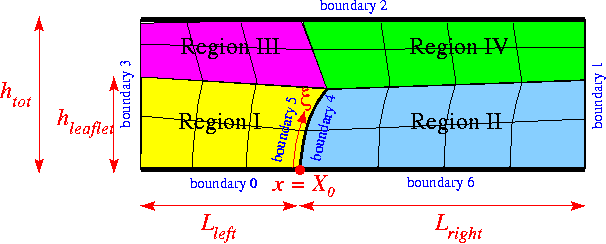Sketch illustrating the algebraic node update procedure.

The implementation of the node update functions is straightforward and can be found in the source files

src/meshes/channel_with_leaflet_mesh.template.h

and

src/meshes/channel_with_leaflet_mesh.template.cc

which also illustrate how the mesh is constructed by inheritance from the SimpleRectangularQuadMesh (the main task being the creation of additional nodes in the interior to "cut open" the mesh along the position of the leaflet). The source files also contain other versions of the mesh in which the node update is performed with Domain/MacroElements, using the technique described in another tutorial.

## Exercise

With the node update strategy illustrated above, the position of all nodes in the fluid mesh has to be updated when the leaflet moves. This is not a particular problem in the current application where the node-update is only performed once per timestep. However, in the corresponding FSI problem , the approach is costly because of the of large number of shape derivatives to be computed.

As an exercise, we suggest to make the node-update procedure more efficient by sub-dividing the regions upstream and downstream of the leaflet into a central section in which the nodes move in response to the motion of the leaflet (the old regions I-IV) and two additional regions (regions V and VI) in which they remain stationary. This is easy because, as explained elsewhere, all AlgebraicNodes already have a default node update function that leaves them stationary.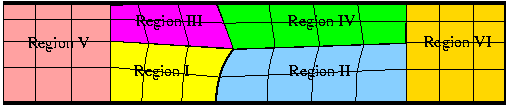A better node update strategy.

# Acknowledgements

• This code was originally developed by Floraine Cordier.